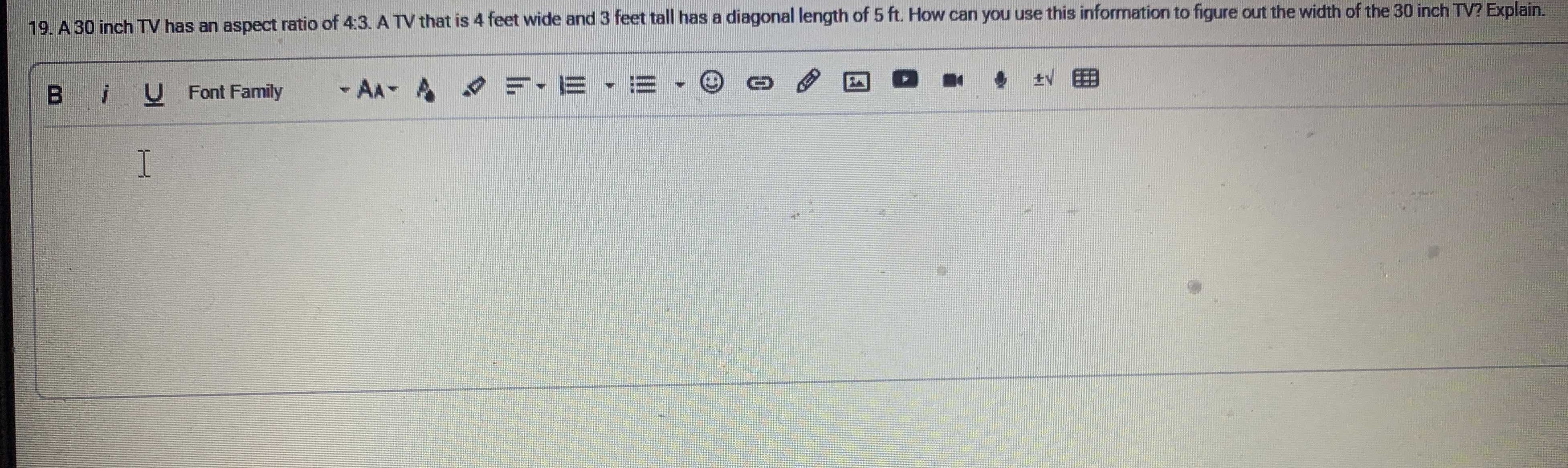### Still have math questions?

Arithmetic
Question

$$30$$ inch TV has an aspect ratio of $$4 : 3$$ . A TV that is $$4$$ feet wide and $$3$$ feet tall has a diagonal length of $$5 ft$$ . t. How can you use this information to figure out the width of the $$30$$ inch TV? Explain.length = $$\frac { 30} { 4} \times 3= 22.5$$ inch